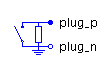Spool Force - MapleSim Help

Spool Force

Component with two horizontal hydraulic connectionsDescription

The Spool Force component models an axial hydraulic back-force exerted on the spool by the hydraulic fluid flowing through the valve.

This component must be connected in series with the spool valve in the circuit where the pressure-flow characteristic is based on the equations of the Orifice component.Variation The Variation parameter selects one of two options that determine how $k$, a coefficient for the spool force, is computed from a look-up table: 1. The table specifies the jet angle $\mathrm{\theta }$ as a function of the displacement; $k$ is the cosine of the jet angle. 2. The table specifies $k$ directly as a function of displacement. The Force Direction parameter specifies the direction of force applied to the spool.Equations $\left\{\begin{array}{cc}\left\{\begin{array}{c}\mathrm{\theta }=\mathrm{MapleSim.Interpolate1D}\left({\mathrm{data}}_{\mathrm{\theta }},\mathrm{displacement},2,\mathrm{Smoothness}\right)\\ k=\mathrm{cos}\left({\mathrm{\theta }}_{\mathrm{converted}}\right)\phantom{\rule[-0.0ex]{3.5ex}{0.0ex}}{\mathrm{\theta }}_{\mathrm{converted}}=\frac{\mathrm{\pi }}{180}\mathrm{\theta }\end{array}\right\}& \mathrm{variation}=1\\ k=\mathrm{MapleSim.Interpolate1D}\left({\mathrm{data}}_{k},\mathrm{displacement},2,\mathrm{Smoothness}\right)& \mathrm{variation}=2\end{array}$ $p={p}_{A}-{p}_{B}\phantom{\rule[-0.0ex]{5.0ex}{0.0ex}}q={q}_{A}=-{q}_{B}$ $A=\mathrm{max}\left(\mathrm{Area},{A}_{\mathrm{min}}\right)$ $F=\mathrm{\rho }\frac{{q}^{2}}{A}k\mathrm{orient}{F}_{\mathrm{dir}}$ ${F}_{\mathrm{dir}}=\left\{\begin{array}{cc}1& \mathrm{Force Direction}=1\\ -1& \mathrm{Force Direction}=2\end{array}$ $\mathrm{orient}=\left\{\begin{array}{cc}1& 0\le q\\ -1& \mathrm{otherwise}\end{array}$ $\mathrm{displacement}=\left\{\begin{array}{cc}1000x& \mathrm{dispUnit1}=1\\ x& \mathrm{otherwise}\end{array}$Variables

 Name Units Description Modelica ID $p$ $\mathrm{Pa}$ Pressure drop from A to B p $q$ $\frac{{m}^{3}}{s}$ Flow rate into port A q $\mathrm{displacement}$ $\mathrm{mm}$ Valve displacement displacement $\mathrm{\theta }$ $\mathrm{degree}$ Jet angle in degrees theta ${\mathrm{\theta }}_{\mathrm{converted}}$ $\mathrm{radian}$ Jet angle in radians theta_convertedConnections

 Name Description Modelica ID $\mathrm{portA}$ Hydraulic port on left side portA $\mathrm{portB}$ Hydraulic port on right side portB $\mathrm{Area}$ Signal input; orifice cross-section in ${\mathrm{mm}}^{2}$ Area $x$ Signal input; valve displacement in $m$ xParametersData Parameters

 Name Default Units Description Modelica ID Variation $1$ Data source variation paramType Data source inline Data source for jet angle as a function of displacement datasourcemode1 ${\mathrm{\theta }}_{x}$ First column: displacement, second column: jet angle table_theta ${\mathrm{data}}_{\mathrm{\theta }}$ data_theta Force direction $1$ Direction of spool force paramType2 Data source inline Data source for k as a function of displacement datasourcemode2 ${k}_{x}$ First column: displacement, second column: discharge coefficient table_k ${\mathrm{data}}_{k}$ data_k $\mathrm{Smoothness}$ linear Smoothness of table interpolation for all the data Smoothness ${\mathrm{\theta }}_{\mathrm{unit}}$ $1$ $\mathrm{degree}$ Unit of jet angle used in the data table dispUnit1 Displacement unit $1$ $\mathrm{mm}$ Unit of displacement used in the data table dispUnit2Fluid Parameters

The following parameters, used in the equations, are properties of the Hydraulic Fluid component used in the model.

 Name Units Description Modelica ID $\mathrm{\nu }$ $\frac{{m}^{2}}{s}$ Kinematic viscosity of fluid nu $\mathrm{\rho }$ $\frac{\mathrm{kg}}{{m}^{3}}$ Density of fluid rho $\mathrm{El}$ $\mathrm{Pa}$ Bulk modulus of fluid El